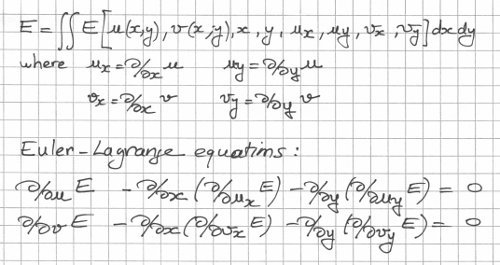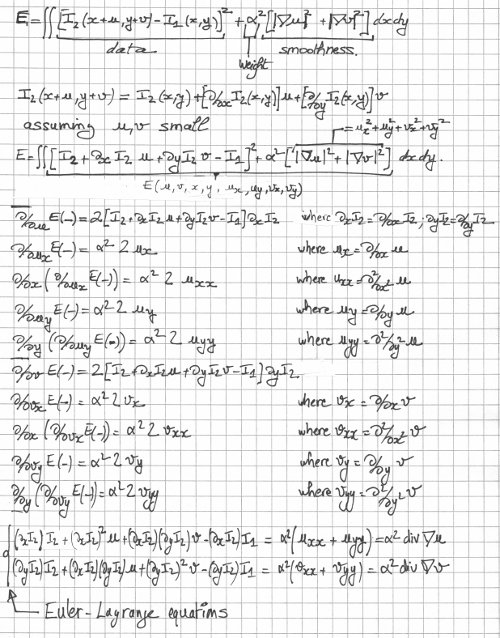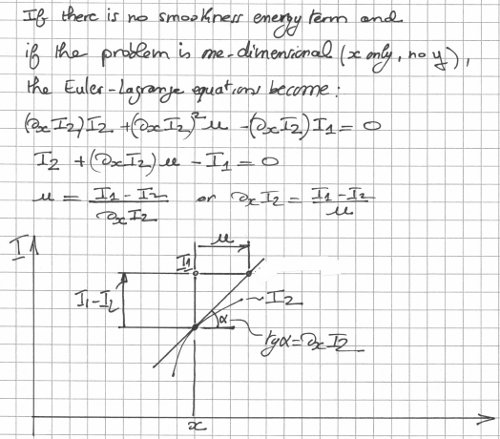## Saturday, June 16, 2012

### Stereo Matching - Variational Methods

Variational methods are global methods in the sense that they are trying to minimize a global energy function made up of two terms, "data" and "smoothness" (also referred to as "prior"). They are called variational methods because the energy is minimized using a mathematical tool called "Calculus of Variations" and in particular the Euler-Lagrange equations. Variational methods have become quite popular in the solution of the optical flow problem, where one estimates the displacement field between two images. Optical flow and stereo correspondence are not that much different. Optical flow is two-dimensional (the displacement field is two-dimensional, that is, objects may move in the x and y directions) while stereo correspondence usually assumes the displacement field is one-dimensional (the disparity is along the x-axis after rectification).

When an energy over a domain needs to be minimized and is of a certain form (see below), the solution of the so-called Euler-Lagrange equations minimizes the energy. Lucky for us, the energies used in optical flow and stereo correspondence are usually of the right type.Euler-Lagrange equations in 2 dimensions.

If I1 is the left image and I2 the right image, if (u,v) represents the displacement, we want: 1) I2(x+u,y+v) to be equal to I1(x,y) for each pixel (x,y) and 2) a smooth displacement field. The following shows how to obtain the Euler-Lagrange equations for the optical flow/stereo matching problem with the assumption that the displacements are small (enables the linearization of the intensity in image 2 and makes the whole thing a lot much easier to solve):Optical flow/stereo matching Euler-Lagrange equations.

At each pixel (x,y), you therefore have 2 equations. The derivatives can be evaluated using finite differences. Each system of 2 equations can be solved quite easily with an iterative scheme.

The process of minimizing the data energy term (for small displacements) can be easily visualized if we consider the problem in one dimension (no y):Physical explanation for the minimization of the data energy term in 1 dimension.

The "smoothness" energy term in the global energy definition is absolutely essential since without it, there would be an infinite number of solutions to the problem. As mentioned in previous posts, the solution of the stereo matching problem is piece-wise smooth, not (fully) smooth.

If the displacements are not small, which is pretty much what you have in real life, the intensity in image 2 can not be linearized the way it is described above. You can still solve the problem without linearizing at that level but it's much more complicated and you have to use a coarse-to-fine strategy. A coarse-to-fine strategy involves the creation of an image pyramid where images are downsampled (reduced in size) until the displacements at the coarsest level are believed to be small. You then solve for the displacements at the coarsest level and take those displacements as the initial state for the next level.

For more information about variational methods in optical flow and stereo matching, I recommend two excellent academic papers on the subject: 1) Horn-Schunck Optical Flow with a Multi-Scale Strategy by Enric Meinhardt-Llopis, Javier Sánchez Pérez, Daniel Kondermann and 2) High Accuracy Optical Flow Estimation Based on a Theory for Warping by Thomas Brox, A. Bruhn, N. Papenberg, J. Weickert